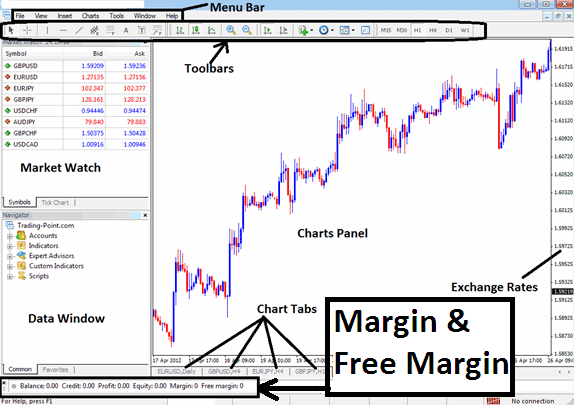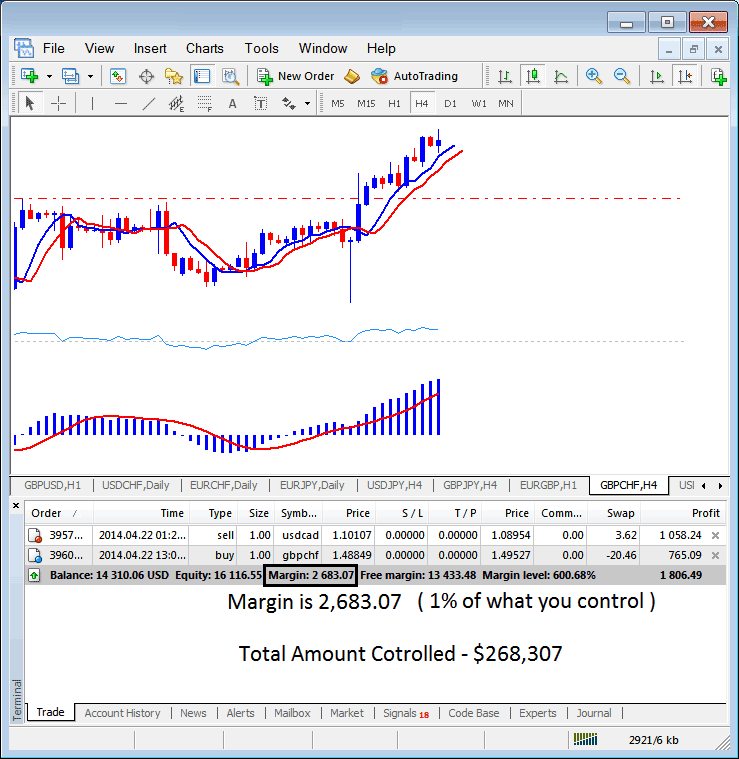# Where to Find Indices Trading Leverage Levels on MT4

Indices Trading Leverage and Margin Levels Shown on MT4Where to Find Indices Trading Leverage Levels on MT4

The indices trading margin example on MT4 indices trading Platform below, the set stock indices leverage is 100:1, the indices trading margin which is 1% is \$2683.07, therefore the total amount controlled by the indices trader is: \$268,307 - this is because with this indices trading leverage the indices trader has used little of his money and borrowed the rest, with this set at 100:1, the indices trader is using 1 % of their trading capital, this 1% is \$2683.07, if 1% is \$2683.07 then 100% is \$268,307Calculating Indices Trading Leverage on MT4 Explained

In the above image example, the indices trader is using \$2683.07, the total controlled amount is \$268,307, but account equity is 16,116.55, therefore used stock indices leverage is (\$268,307 divide by 16,116.55) = 16.64 : 1

16.64 : 1

In the indices trading account above the Used indices trading leverage is 16.64 : 1

Start Trading Crypto CFDs with a \$30 Bonus